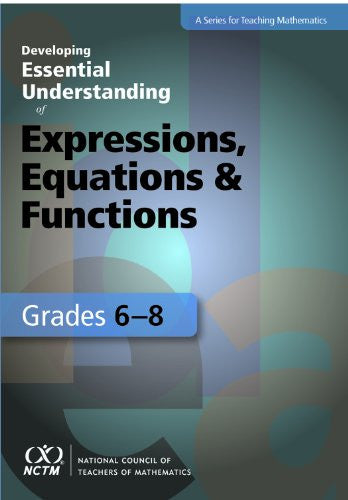Math Resources and Games# Developing Essential Understanding of Expressions, Equations & Functions Grades 6-8

\$36.95

Author: Gwendolyn Lloyd

Edition: First

ISBN: 0873536703

Number Of Pages: 116

Publisher: National Council of Teachers of Mathematics

Details: Why do some equations have one solution, other two or even more solutions, and some no solutions? Why do we sometimes need switch the direction of an inequality symbol in solving an inequality? What could you say if a student described a function as an equation? How much do you know...and how much do you need to know? Helping your students develop a robust understanding of expressions, equations, and functions requires that you understand this mathematics deeply. But what does that mean? This book focuses on essential knowledge for teachers about expressions, equations, and functions. It is organized around five big ideas, supported by multiple smaller, interconnected ideas essential understandings.Taking you beyond a simple introduction to expressions, equations, and functions, the book will broaden and deepen your mathematical understanding of one of the most challenging topics for students and teachers. It will help you engage your students, anticipate their perplexities, avoid pitfalls, and dispel misconceptions. You will also learn to develop appropriate tasks, techniques, and tools for assessing students' understanding of the topic. Focus on the ideas that you need to understand thoroughly to teach confidently.